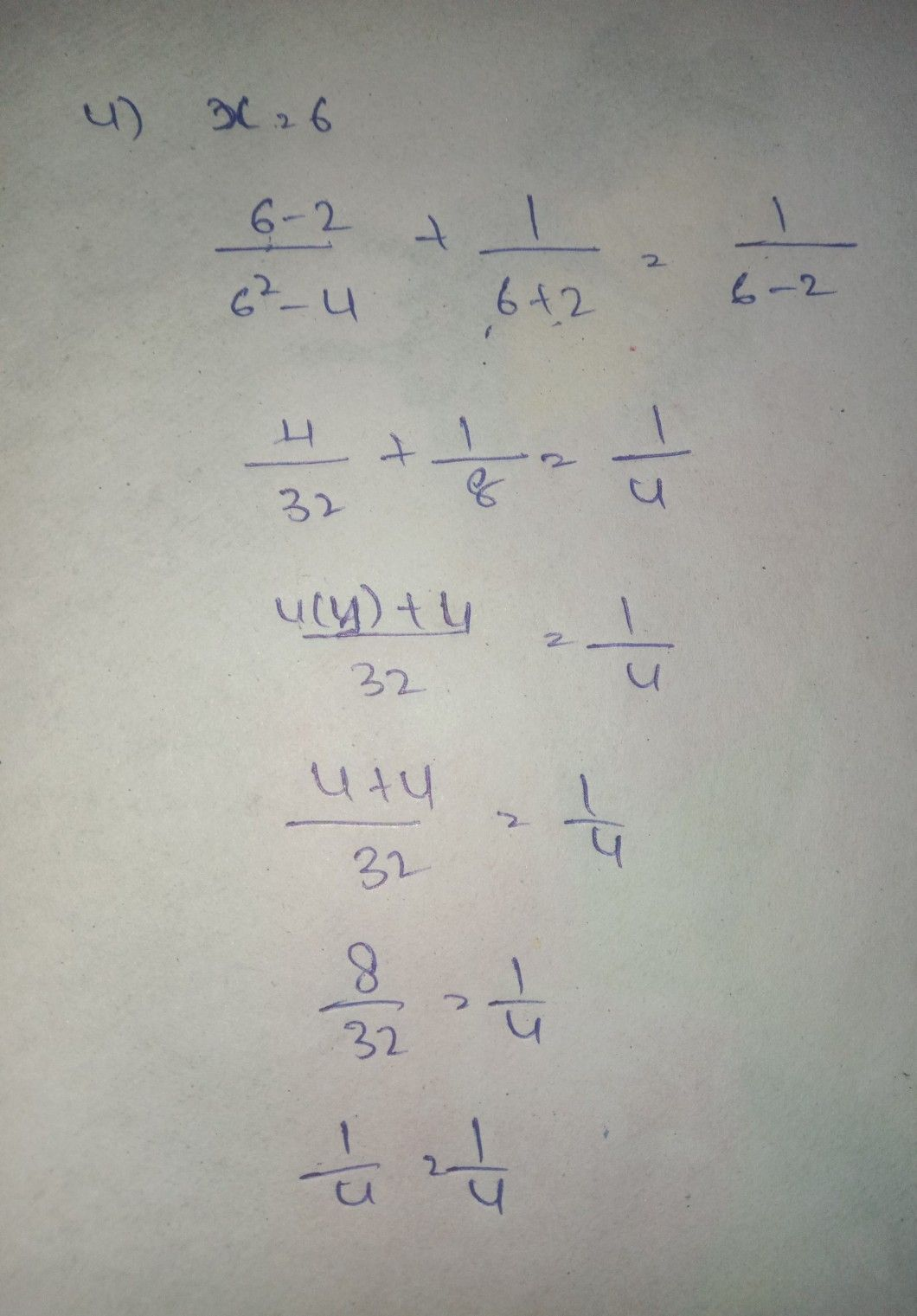Symbol
ProblemActivity $1.$ Solve the following rational equations and inequalities using the guided procedure on the table below 1. Rational Equation $\dfrac {x-2} {x^{2-4}}+\dfrac {1} {x+2}=\dfrac {1} {x-2}$ $1$ Find the Least Common Denominator $\left(1CD\right)$ $2$ Multiply both sides of the equation by its the LCD. $3$ Apply the Distributive Property and then simplify. $4$ Find all the possible values of $x$ $x=6$ $5$ Check each value by substituting into the original equation and reject any extraneous $0ot$
10th-13th grade
Calculus
Search count: 106
SolutionQanda teacher - kattalaharStudent
Thank you so much, maam! ❤️